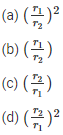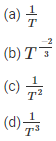# Gravitation MCQs for NEET

NEET  Physics is the scoring paper in the medical entrance examination. Here, you will discover the NEET Physics MCQ Questions for all Concepts as per the latest syllabus. Practice more on a regular basis with these NEET Physics objective questions on air pollution and improve your subject knowledge & problem-solving skills along with time management. NEET Physics Gravitation Multiple Choice Questions make you feel confident in answering the question in the exam & increases your scores to high.

## MCQ on Gravitation

1. What happens to the gravitational potential at the centre of the uniform spherical shell which shrinks gradually?
(a) Remains constant
(b) Decreases
(c) Increases
(d) Oscillates

2. What is the relation between the escape velocity and orbital velocity of a satellite, if the satellite is close to the earth’s surface?Answer: (b) $$v_{e}=\sqrt{2} v_{0}$$

3. What happens to the weight of the body if the weight becomes $$\frac{1}{16}$$ at a certain. Also, consider the radius of the earth to be R.
(a) 4R
(b) 15R
(c) 5R
(d) 3R

4. What is the maximum height attained by the rocket above the surface of the earth if the mass of the rocket is M, and the initial speed to be V. Assume that R is the radius of the earth.Answer: (b) $$\frac{R}{\frac{2 g R}{v^{2}}-1}$$

5. What is the increase in the potential energy of the body with mass m if the body is taken at the height h which is equal to the radius of the earth?
(a) mgR
(b) 2mgR
(c) $$\frac{1}{2}$$mgR
(d) $$\frac{1}{4}$$mgR

Answer: (c) $$\frac{1}{2}$$mgR

6. Consider a body of mass m which needs to be moved from an orbit of radius 2R to 3R. What is the energy requiredAnswer: (b) $$\frac{G M m}{6 R}$$

7. The distance between the closest planet and the sun is r1 while the distance between the farthest planet and the sun is r2. The linear velocities of these planets is v1 and v2 respectively. What is the ratio of $$\frac{v_{1}}{v_{2}}$$Answer: (c) $$\left(\frac{r_{2}}{r_{1}}\right)$$

8. Choose the factor on which the orbital velocity does not depend when the satellite is orbiting close to the earth’s surface
(a) The mass of the earth
(b) The mass of the satellite
(d) The radius of the earth

Answer: (b) The mass of the satellite

9. If a satellite takes time T for revolution, the kinetic energy is proportional toAnswer: (b) $$T^{\frac{-2}{3}}$$